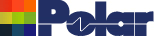Rounding loss tangent leads to large modeling errors Application Note AP8194 Rounding loss tangent leads to large modeling errors Because Loss Tangent (TanD) is a small number it is perhaps easy to forgive people who round it off to fewer decimal places. Our minds are wired to dismiss numbers far to the right of the decimal point. However, this can lead to unintended miscalculations when rounding small value parameters such as Loss Tangent which has a directly proportional effect on the insertion loss. A rounding to 2 decimal places of a TanD say, from 0.015 to 0.02 – quite legitimate, you may think – would actually lead to the modeling of insertion loss being over predicted by a massive 33%. Using the Si9000e PCB field solver, from the Si9000e structure bar select the Offset Stripline 1B1A controlled impedance structure.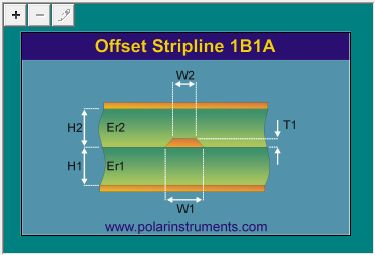Supply the structure parameters and goal seek for an impedance of 50 ohms.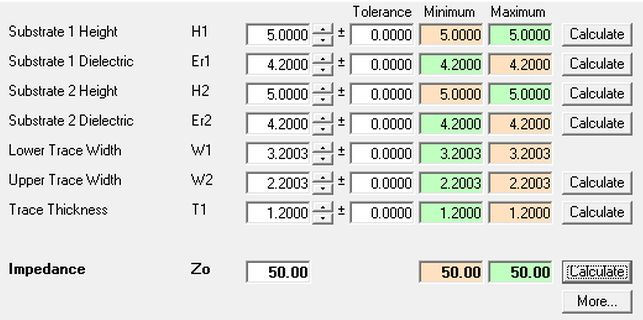Switch to the Frequency Dependent Calculation tab and specify a frequency range of 500MHz – 20GHz and a value of 0.015 for Loss Tangent (TanD). From the Surface Roughness Compensation dialog choose Smooth.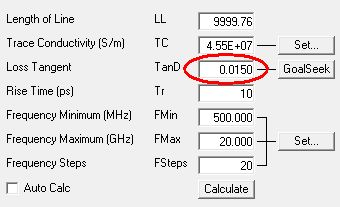Click Calculate and display the losses against frequency; (you can also click the chart at the 20GHz data point on the Dielectric Loss series to display its value) – in this case, a loss value of –14.24 dB.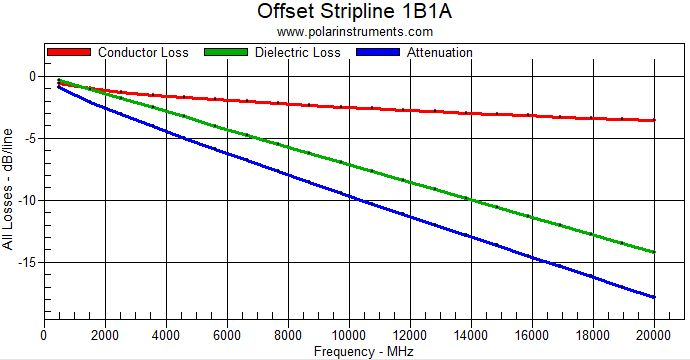Rounding the value of Loss Tangent How will the value of Dielectric Loss change if the value of Loss Tangent is rounded from 0.015 to 0.02? Change the value of the Loss Tangent to 0.02.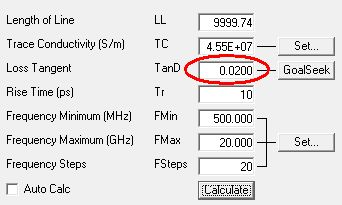Click Calculate and and note the new loss value of –18.98 dB at the 20GHz data point on the Dielectric Loss series .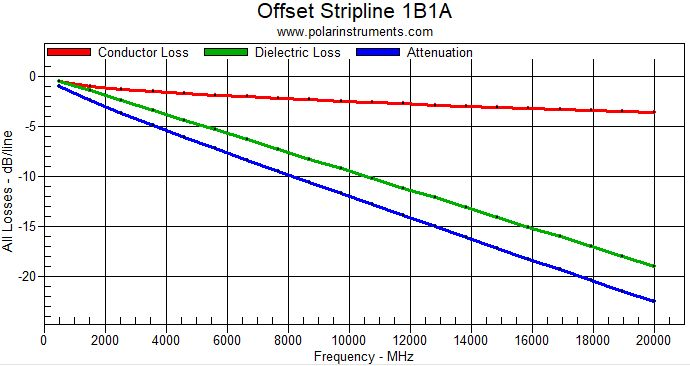Rounding from 0.015 to 0.02 actually represents a change (increase) in Loss Tangent value of 33% This increase in turn results in a corresponding increase (shown below) in Dielectric Loss of 33%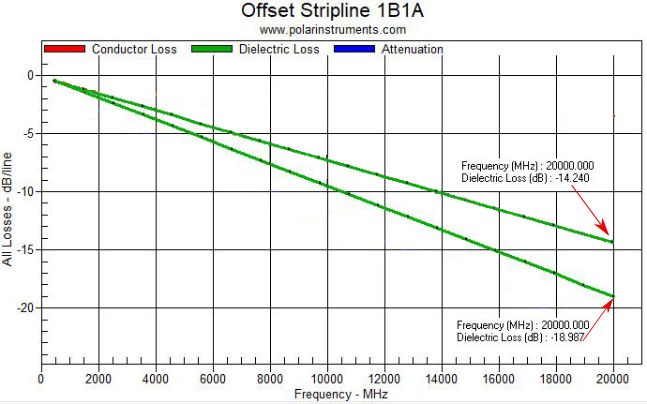It may be easier to visualize the real difference in Loss Tangent (and avoid unexpected errors) if the values are expressed in engineering notation. The actual value of Loss Tangent, 0.015, is expressed in engineering notation as 15 x 10–3, but the rounded value, 0.02, is expressed as 20 x 10–3, an obvious increase of 33%.